# Credit Card Fraud Detection: Solve the FinTech puzzle with AI

Due to the recent advancement in technology, more and more people are using credit cards. And not only people but every organization, from small-sized businesses to big enterprises, are using credit cards as their mode of payment. And this advancement is ultimately breeding to scams like Credit Card Fraud as the other side of the coin.

Credit Card Fraud is one of the biggest scams faced by many government agencies and big companies, with an enormous amount of money involved in these transactions. So, it needs some solution to deal with the loss of billions of dollars.

This can be achieved using Machine Learning that can instantly recognize a fraudulent transaction, and it can at least save some amount of money involved. However, there are many challenges faced by many service providers while developing a solution using AI in finance problems.

In this blog, we will be looking at the problem that will arise due to the highly imbalanced data, their probable solution, and the step-by-step coding method for credit fraud detection. We will be using the Kaggle dataset in Google Colab for the fraud detection solution with supervised learning using AI & Machine Learning.

### Have a look at some of the problems:

• The model training in supervised learning requires good quality data. However, due to the privacy policies of the banks in place, they cannot share the data in its direct form for training which raises the issue of Data Availability.
• Even though we gain a quality dataset, not violating any of the privacy policies, the dataset would be Highly Imbalanced and thus making it tough to identify the fraudulent transactions from the authentic ones.
• Also, the AI model must be fast enough to identify a fraudulent transaction which can be a challenge since Enormous Data is processed every day.
• Last but not least, scammers may invent new techniques in which the model cannot detect these fraudulent transactions.

### Some solutions to tackle these challenges:

• To deal with the data availability issue, the Dimensionality of the Dataset can be reduced. Methods like LDA(Linear discriminant analysis), PCA(Principal Component Analysis), etc., can be used in achieving the said target.
• A highly imbalanced dataset can be converted into a balanced dataset using Resampling Methods like class weights, Random Oversampling or Undersampling, a combination of SMOTE and Tomek Links, etc.
• The model used must be simple and fast enough to handle the enormous data and can be at the cost of minimal accuracy.
• If the built model is simple enough, we can apply changes with some tweaks and deploy a whole new model to avoid any new techniques used by scammers against the model.

### METHOD AND CODE:

First of all, choose a coding platform to code the model. We have used Google Colab as the platform, which is well suited for machine learning. However, there is no restriction in using Kaggle or any other coding platform of your convenience. You can also use Jupyter Notebook to code on your local machine rather than using cloud-based platforms.

#### Installing Important Dependencies:

Listed below are all the dependencies needed:

``````
import numpy as np
import pandas as pd
import matplotlib.pyplot as plt
import seaborn as sns
``````

The above code will import all the libraries we will use if we run this code cell in Google Colab or Kaggle. However, you will have to install these libraries separately in your system if you are working on your local machine, after which the above code cell will import these libraries.

#### Dataset:

The dataset used here is taken from the Kaggle website and can be downloaded by clicking on the download button on the top-right corner of the website here.

After downloading the Kaggle dataset, use the below cell to load the data into the ‘data’ variable by specifying the path location of the dataset.

``````
``````

NOTE: The path provided in parentheses must be the same as the path location of your Kaggle dataset. If you use Google Colab, you have two options: either upload the dataset or mount your Google Drive to fetch the data.

``````
drive.mount('/content/gdrive')
``````

#### Understanding the data:

Let us take a look at our data: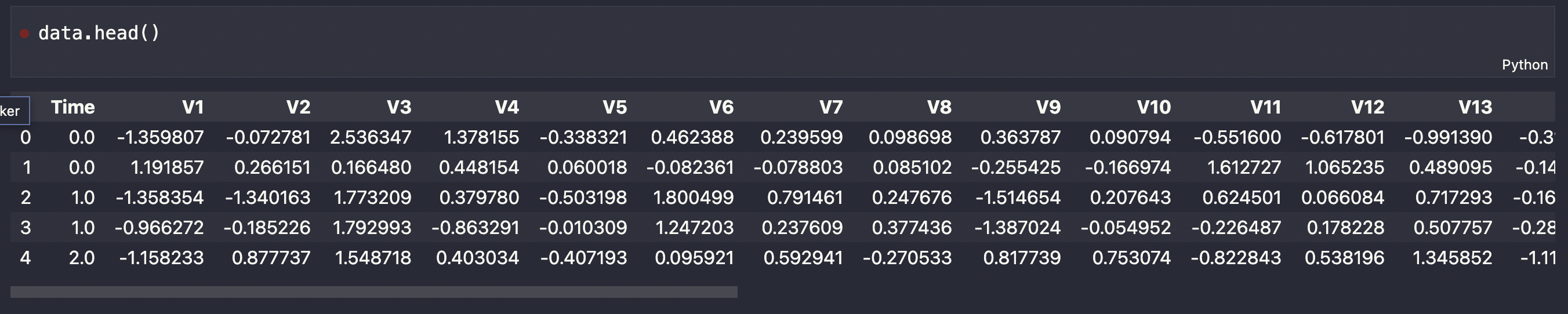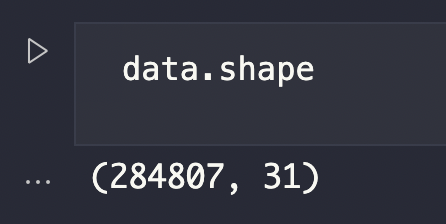The dataset contains 28 original feature columns, which are replaced with V1, V2, …, V28 using PCA transformation for the privacy of the user. The other three additional columns are time, amount, and class.

Now, let us observe what are the features of the data by executing the given code.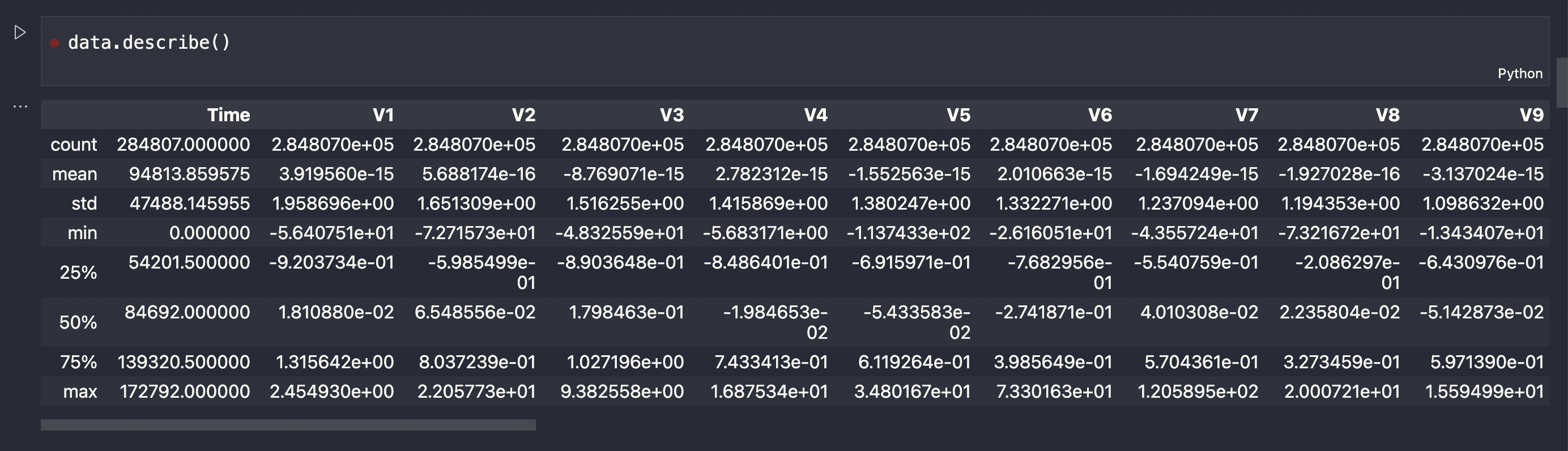We can see features like total count, mean, standard deviation, minimum, maximum, etc., for all the columns.

The time column contains the time elapsed between the first transaction and the current one. To obtain more knowledge, visualize it using the Seaborn library. We can see here that the density of fraud transactions is usually more at night when compared to the authentic ones, which are less by looking at the graph as shown below.

``````
plt.figure(figsize=(14,8))
sns.distplot(data['Time'][data['Class']==0], bins=25, label='Not Fraud')
sns.distplot(data['Time'][data['Class']==1], bins=25, label='Fraud')
plt.legend()
``````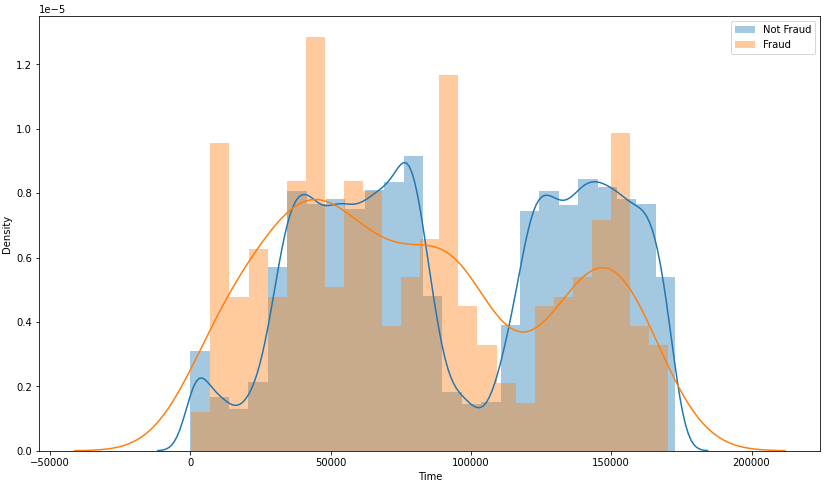We can similarly visualize the amount column as well. Here, we can see that the density of low amounts is the highest, and only some of them are high amount transactions. The same can also be inferred from observing the mean value, which is only \$88, and the highest amount is \$25691, indicating that the distribution is heavily right-skewed.

``````
plt.figure(figsize=(14,8))
sns.distplot(data['Amount'], bins=25)
``````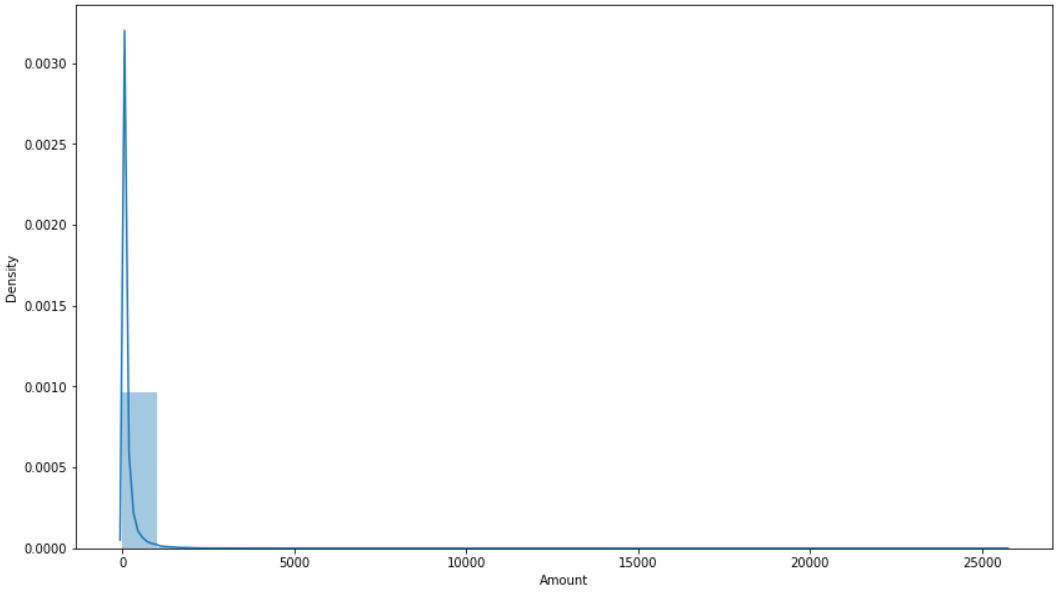#### Class Distribution:

Now let’s see the total number of fraudulent and non-fraudulent transactions and compare them.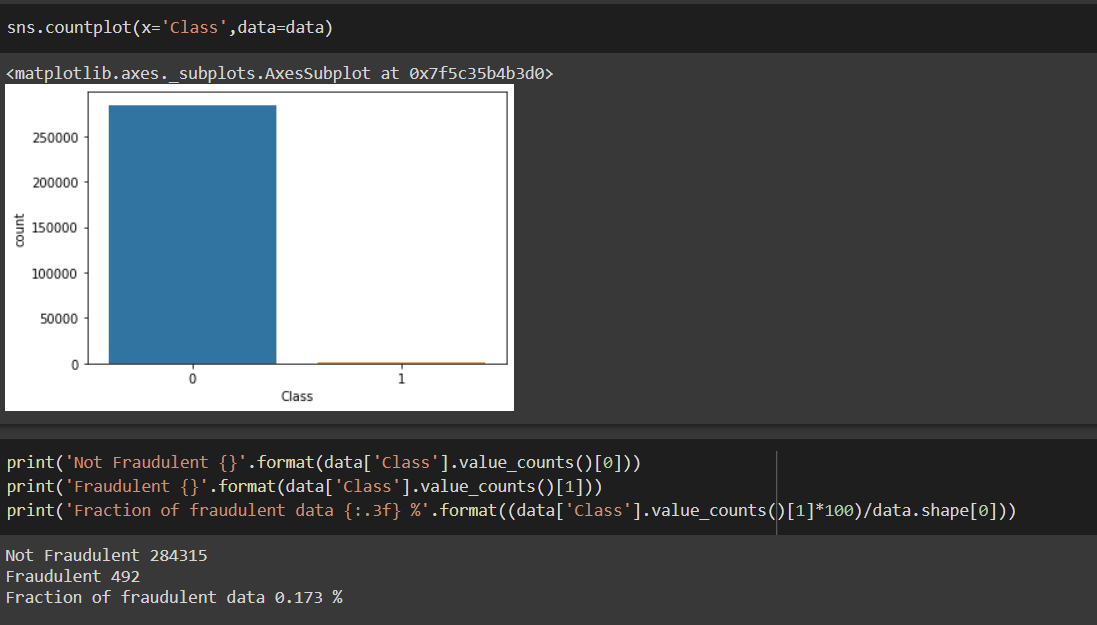As we can see, only 0.173% of the whole data are fraudulent cases which are less to train our model and the reason why we cannot solely depend on accuracy as our only metric for the result. We must use some resampling techniques to get our model to learn more about fraudulent cases.

Using the correlation matrix, we can get some insight into what is the correlation between all the features. This correlation will help us to know which features we can use for prediction.``````
plt.figure(figsize=(10,10))
sns.set_context('paper', font_scale=1.4)

data_corr= data.corr()
sns.heatmap(data_corr)
``````

Now, you may ask why do we need fraud analytics if only a small amount of fraudulent transactions occur. Well, to put it simply, fraudulent transactions happen in billions of dollars every year, and if we save only 0.1% of these transactions will mean we have saved millions of dollars. This is not a small amount considering that it can change the lives of many people.

#### Outlier Removal using IQR(Interquartile Range):

Outlier removal can be a difficult task, as the trade-off between reducing the number of transactions and the amount of information left is not easily solvable and it depends on the amount of data as well. This method says that the data outside the 1.5 times IQR is usually considered as outliers. However, if we take 1.5*IQR, then it decreases our dataset size drastically, and hence we have used 1*IQR instead of 1.5*IQR to balance the trade-off.

``````
Q1 = X.quantile(0.25)
Q3 = X.quantile(0.75)
IQR = Q3-Q1
print(IQR)
``````
``````
X_final = X[((X < (Q1 - 1 * IQR)) |(X> (Q3 + 1 * IQR))).any(axis=1)]
print(X_final.index)
``````

And now the amount of data left is:

``````
Y=data.drop(index=X_out.index)['Class']
Y.value_counts()
``````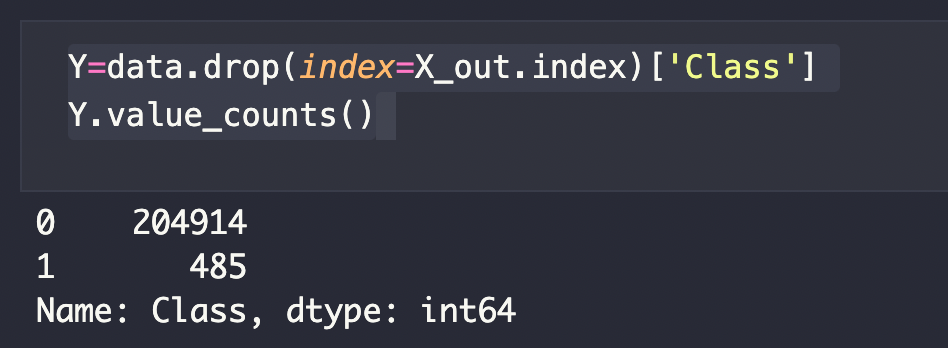#### Splitting the Dataset into Training and Testing data:

In order to make our model learn, we need to split the Kaggle dataset that we have downloaded into two parts viz. training dataset and testing dataset. The training dataset will train the model with the data such that when the testing dataset is being provided as the input, the AI model can analyze and give the desired output.

Scikit Learn library provides such preinstalled tool to split the dataset, the code cell for the same is as below:

‘train_test_split’ from scikit learn library is used here.

``````
from sklearn.model_selection import train_test_split
X_train, X_test, Y_train, Y_test= train_test_split(X_final, Y, test_size=0.2, random_state=69)
``````

#### Resampling data using SMOTE-Tomek Links:

By only using Random Undersampling or Oversampling, it causes a reduction in data size and increment in data size, which then increases training time. And since our model needs to be simple and fast enough, we have used a combination of SMOTE, which oversamples the minority class, and Tomek Links, which removes the examples from the majority class, to produce a balanced distribution.

``````
from collections import Counter
from imblearn.combine import SMOTETomek
X_sampled_train, Y_sampled_train= smoteenn.fit_resample(X_train, Y_train)
print(Counter(Y_sampled_train))
``````
``````
Counter({1: 163927, 0: 163193})
``````

As we can see the class distribution is very balanced right now.

#### Building Classifier models to predict the results:

##### 1. Decision Tree Classifier:
``````
from sklearn.tree import DecisionTreeClassifier
model= DecisionTreeClassifier(random_state=69, criterion='entropy')
model.fit(X_sampled_train, Y_sampled_train)
``````
``````
from sklearn.metrics import confusion_matrix, accuracy_score, precision_score, recall_score, f1_score, roc_auc_score
Y_pred_train= model.predict(X_sampled_train)
Y_pred_dt= model.predict(X_test)
print('Accuracy and cm of training set: ')
print('Confusion Matrix:\n', confusion_matrix(Y_sampled_train,Y_pred_train))
print('Accuracy Score', accuracy_score(Y_sampled_train,Y_pred_train))
print('Accuracy and cm of test set: ')
print('Confusion Matrix:\n', confusion_matrix(Y_test,Y_pred_dt))
print('Accuracy Score', accuracy_score(Y_test,Y_pred_dt))
print('Precision Score', precision_score(Y_test,Y_pred_dt))
print('Recall Score', recall_score(Y_test,Y_pred_dt))
print('F1 Score', f1_score(Y_test,Y_pred_dt))
print('ROC AUC Score', roc_auc_score(Y_test,Y_pred_dt))
``````
``````
Accuracy and cm of training set:
Confusion Matrix:
[[163193 0]
[ 0 163927]]
Accuracy Score 1.0
Accuracy and cm of test set:
Confusion Matrix:
[[40916 71]
[ 13 80]]
Accuracy Score 0.9979552093476144
Precision Score 0.5298013245033113
Recall Score 0.8602150537634409
F1 Score 0.6557377049180327
ROC AUC Score 0.9292413985971425
``````

As we can see in the decision tree classifier, there is a good amount of accuracy and recall, however, the precision score is not satisfactory. And this can also be inferred from the confusion matrix where false-positive transactions are more than they should be.

##### 2. Random Forest Classifier:
``````
ffrom sklearn.ensemble import RandomForestClassifier
model= RandomForestClassifier(random_state=69)
model.fit(X_sampled_train, Y_sampled_train)
``````
``````
from sklearn.metrics import confusion_matrix, accuracy_score, precision_score, recall_score, f1_score, roc_auc_score
Y_pred_train= model.predict(X_sampled_train)
Y_pred_rf= model.predict(X_test)
print('Accuracy and cm of training set: ')
print('Confusion Matrix:\n', confusion_matrix(Y_sampled_train,Y_pred_train))
print('Accuracy Score', accuracy_score(Y_sampled_train,Y_pred_train))
print('Accuracy and cm of test set: ')
print('Confusion Matrix:\n', confusion_matrix(Y_test,Y_pred_rf))
print('Accuracy Score', accuracy_score(Y_test,Y_pred_rf))
print('Precision Score', precision_score(Y_test,Y_pred_rf))
print('Recall Score', recall_score(Y_test,Y_pred_rf))
print('F1 Score', f1_score(Y_test,Y_pred_rf))
print('ROC AUC Score', roc_auc_score(Y_test,Y_pred_rf))
``````
``````
Accuracy and cm of training set:
Confusion Matrix:
[[163193 0]
[ 0 163927]]
Accuracy Score 1.0
Accuracy and cm of test set:
Confusion Matrix:
[[40977 10]
[ 12 81]]
Accuracy Score 0.9994644595910419
Precision Score 0.8901098901098901
Recall Score 0.8709677419354839
F1 Score 0.8804347826086956
ROC AUC Score 0.9353618810685056
``````

As we can see, the random forest classifier has accuracy, precision, and recall values that are quite a satisfactory one with an F1 score of 0.88 and ROC AUC score of 0.935.

#### Conclusion:

At last, we can witness that by using machine learning models, we can save people’s money from many fraudulent transactions and that too easily and very fast. Moreover, the privacy of the customers has been kept intact, and the problem of the imbalanced dataset is also resolved by analyzing the fraud analytics. So all the challenges which were discussed above are almost dealt with using the supervised learning model of machine learning.

Also, for more accurate results, you can amend these methods and include many techniques like:

• Hyperparameter Tuning: Hyperparameter can be tuned with the help of GridSearchCV or RandomizedSearchCV to improve results.
• Use Feature Scaling for other algorithms like Logistic Regression, XGBoost, SVM, etc which may perform better than Random Forest.
• One can also use the stacking of different models to get more accurate results.

Get hands-on with the above techniques to solve the puzzle. Feel free to reach out to us in case you find any struggle. We, at Seaflux, are AI & Machine Learning enthusiast who is helping enterprises worldwide. Have a query or want to discuss AI or Machine Learning projects? Schedule a meeting with us here, we'll be happy to talk to you!##### Jay Mehta

Director of Engineering